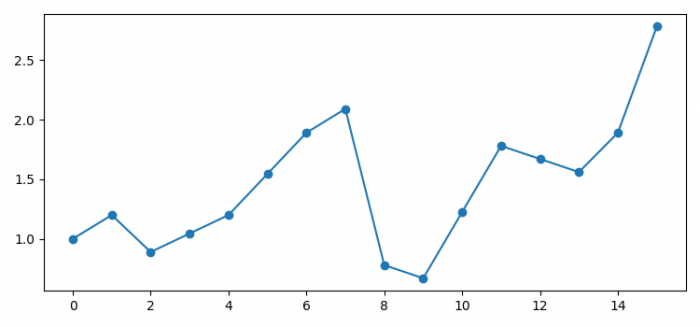# Remove NaN values from a dataframe without fillna or Interpolate (Python Matplotlib)

To remove NaN values from a dataframe without filter or interpolate, we can take the following steps −

## Steps

• Set the figure size and adjust the padding between and around the subplots.

• Create an array to make a Pandas data frame.

• One-dimensional ndarray with axis labels (including time series).

• Plotting interpolation, 'index', 'values' − Use the actual numerical values of the index.

• To display the figure, use show() method.

## Example

import numpy as np
import pandas as pd
from matplotlib import pyplot as plt

# Set the figure size
plt.rcParams["figure.figsize"] = [7.50, 3.50]
plt.rcParams["figure.autolayout"] = True

# Numpy array
data = np.array([1., 1.2, 0.89, np.NAN,
1.2, np.NAN, 1.89, 2.09,
.78, .67, np.NAN, 1.78,
np.NAN, 1.56, 1.89, 2.78]
)

# Pandas dataframe
df = pd.Series(data)

# Plot the interpolation
df.interpolate('index').plot(marker='o')

# Display the plot
plt.show()

## Output

It will produce the following output −